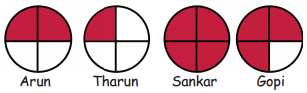Tamilnadu State Board New Syllabus Samacheer Kalvi 4th Maths Guide Pdf Term 3 Chapter 6 Fraction InText Questions Textbook Questions and Answers, Notes.

## Tamilnadu Samacheer Kalvi 4th Maths Solutions Term 3 Chapter 6 Fraction InText Questions

In simple terms, this fraction and whole number calculator allows you to solve fraction problems with whole numbers and fractions form.

Activity (Text Book Page No. 51)

(The four equal parts of a circle)
A Circle which is divided into four equal parts are given to Arun, Tharun, sankar and Gopi, to colour it.
They could complete the task of colouring in two minutes, which is given below.In the above pictures one fourth (quarter) portion was coloured by tharun.
1/2 portion was coloured by __________
Arun

Whole portion was coloured by __________
Sankar

Three fourth of the portion was coloured by __________
GopiCan we try this: (Text Book Page No. 55)

Question 1.
Anitha’s rectangular garden was divided into ________ parts.
4

Question 2.
Portion of brinjal planted part = ________
$$\frac { 1 }{ 4 }$$
$$\frac { 2 }{ 1 }$$$$\frac { 1 }{ 4 }$$